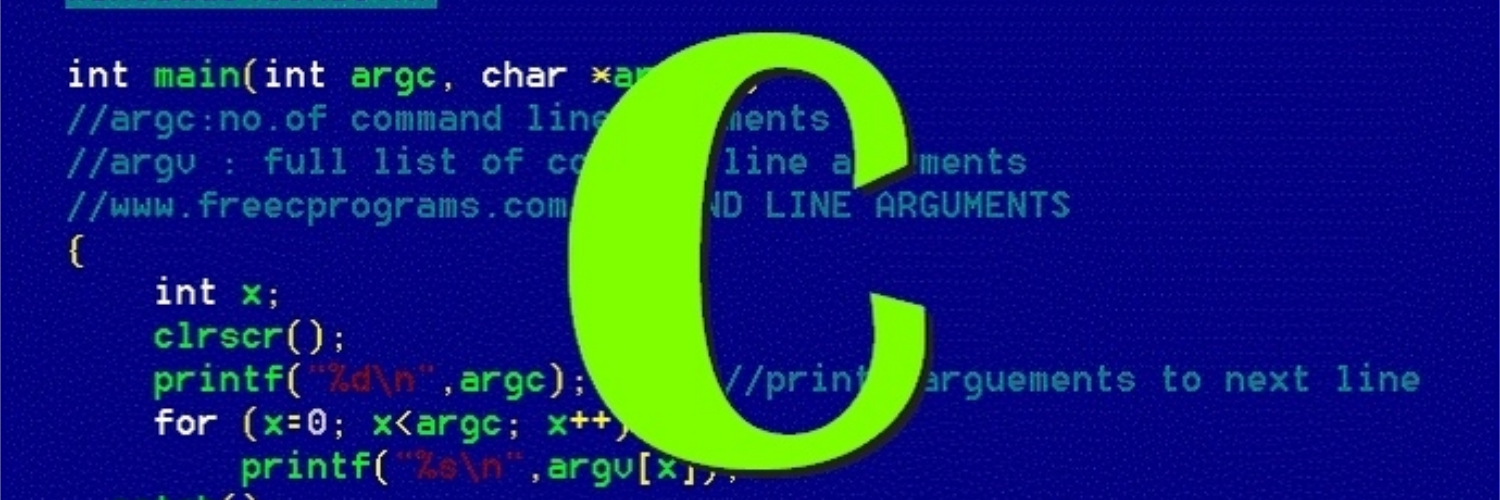# Practical C - Stack

Implement Stack in CIn this blog post, let’s implement a generic stack in C.

We will do this by several iterations, from simple version to the full blown version step by step.

# An Integer Version

#include <assert.h>
#include <stdio.h>
#include <stdlib.h>

typedef struct {
// int array to store elements of the stack
int *elems;

// the actual length of the stack
int logicalLen;

// the allocated length of the array
int allocLen;
} stack;

// Initialize a stack
void StackNew(stack *s);

// Dispose a stack
void StackDispose(stack *s);

// Push an element onto the stack
void StackPush(stack *s, int value);

// Pop an element from the stack
int StackPop(stack *s);

void StackNew(stack *s) {

// allocate 4 elements for the array
s->elems = (int *)malloc(4 * sizeof(int));
s->allocLen = 4;
s->logicalLen = 0;
}

void StackDispose(stack *s) { free(s->elems); }

void StackPush(stack *s, int value) {
if (s->logicalLen == s->allocLen) {
// double the size of the array if there is no free space in it
s->elems = (int *)realloc(s->elems, 2 * s->allocLen * sizeof(int));
s->allocLen *= 2;
}
s->elems[s->logicalLen] = value;
s->logicalLen++;
}

int StackPop(stack *s) {
assert(s->logicalLen != 0);
int popped = s->elems[s->logicalLen - 1];
s->logicalLen--;
return popped;
}

void PrintStack(stack *s) {
for (int i = 0; i < s->logicalLen; i++) {
printf("%d ", s->elems[i]);
}
printf("\n");
}

int main() {
stack s;
int popped;

StackNew(&s);
StackPush(&s, 1);
StackPush(&s, 2);
StackPush(&s, 3);
StackPush(&s, 4);
StackPush(&s, 5);
PrintStack(&s);
popped = StackPop(&s);
printf("%d popped!\n", popped);
popped = StackPop(&s);
printf("%d popped!\n", popped);
popped = StackPop(&s);
printf("%d popped!\n", popped);
popped = StackPop(&s);
printf("%d popped!\n", popped);
popped = StackPop(&s);
printf("%d popped!\n", popped);
//popped = StackPop(&s); // error
//printf("%d popped!", popped);
StackDispose(&s);
}

# A Generic Version

We don’t want to constrain to only Integer, let’s have a generic version that takes any type.

#include <assert.h>
#include <stdio.h>
#include <stdlib.h>
#include <string.h>

typedef struct {
void *elems;
// how many bytes each element takes
int elemSize;
int logicalLen;
int allocLen;
} stack;

void StackNew(stack *s, int elemSize);
void StackDispose(stack *s);
void StackPush(stack *s, void *value);
void StackPop(stack *s, void *popped);

void StackNew(stack *s, int elemSize) {
s->elems = malloc(4 * elemSize);
s->elemSize = elemSize;
s->allocLen = 4;
s->logicalLen = 0;
}

void StackDispose(stack *s) { free(s->elems); }

void StackPush(stack *s, void *value) {
if (s->logicalLen == s->allocLen) {
s->elems = realloc(s->elems, 2 * s->allocLen * s->elemSize);
s->allocLen *= 2;
}
memcpy((char *)s->elems + s->logicalLen * s->elemSize, value, s->elemSize);
s->logicalLen++;
}

void StackPop(stack *s, void *popped) {
assert(s->logicalLen != 0);
memcpy(popped, (char *)s->elems + (s->logicalLen - 1) * s->elemSize,
s->elemSize);
s->logicalLen--;
}

void PrintStack(stack *s) {
for (int i = 0; i < s->logicalLen; i++) {
printf("%d ", *((char *)s->elems + i * s->elemSize));
}
printf("\n");
}

int main() {
stack s;
int popped;

StackNew(&s, sizeof(int));

int n1 = 1;
StackPush(&s, &n1);

int n2 = 2;
StackPush(&s, &n2);

int n3 = 3;
StackPush(&s, &n3);

int n4 = 4;
StackPush(&s, &n4);

int n5 = 5;
StackPush(&s, &n5);

PrintStack(&s);

StackPop(&s, &popped);
printf("%d popped!\n", popped);

StackPop(&s, &popped);
printf("%d popped!\n", popped);

StackPop(&s, &popped);
printf("%d popped!\n", popped);

StackPop(&s, &popped);
printf("%d popped!\n", popped);

StackPop(&s, &popped);
printf("%d popped!\n", popped);

StackDispose(&s);
}

The differences in the generic version is of course the lack of type information. You can see that in places that we try to read or write, we have to count the bytes directly.

# An Inproved Version

By far, to push elements onto the stack, we copy the memory bit pattern directly into the stack’s memory space. If the stack contains int or char this is no problem, but what if the elements themselves are pointers?

True the pointers will be copied over, and the DisposeStack function should also free the contents that these pointers point to. Why so? OK, let me explain. Let’s say you have a thing, and you push it onto some kind of stack, who now owns the thing? The stack. This means the ownership of the thing transfers from you the stack. And when the thing gets popped back, the ownership transfers back to you again. Because of this, when you try to dispose the stack, all the elements on the stack have to be completely cleared. That is to say that if they are pointers, we have to free the contents they point to before we free the elements in the stack.

The solution is to add a free function that knows how to truely free each element in stack.

#include <assert.h>
#include <stdio.h>
#include <stdlib.h>
#include <string.h>

typedef struct {
void *elems;
// how many bytes each element takes
int elemSize;
int logicalLen;
int allocLen;
void (*freeFn)(void *p);
} stack;

void StackNew(stack *s, int elemSize, void (*freeFn)(void *p));
void StackDispose(stack *s);
void StackPush(stack *s, void *value);
void StackPop(stack *s, void *popped);

void StackNew(stack *s, int elemSize, void (*freeFn)(void *p)) {
s->elems = malloc(4 * elemSize);
s->elemSize = elemSize;
s->allocLen = 4;
s->logicalLen = 0;
s->freeFn = freeFn;
}

void StackDispose(stack *s) {
if (s->freeFn != NULL) {
for (int i = 0; i < s->logicalLen; i++) {
s->freeFn((char *)s->elems + i * s->elemSize);
}
} else {
free(s->elems);
}
}

void StackPush(stack *s, void *value) {
if (s->logicalLen == s->allocLen) {
s->elems = realloc(s->elems, 2 * s->allocLen * s->elemSize);
s->allocLen *= 2;
}
memcpy((char *)s->elems + s->logicalLen * s->elemSize, value, s->elemSize);
s->logicalLen++;
}

void StackPop(stack *s, void *popped) {
assert(s->logicalLen != 0);
memcpy(popped, (char *)s->elems + (s->logicalLen - 1) * s->elemSize,
s->elemSize);
s->logicalLen--;
}

void PrintStack(stack *s) {
for (int i = 0; i < s->logicalLen; i++) {
printf("%s ", *((char **)s->elems + i));
}
printf("\n");
}

void freeString(void *p) { free(*(char **)p); }

int main() {
const char *friends[] = {"Bob", "Jane", "Wang"};

stack stringStack;

StackNew(&stringStack, sizeof(char *), freeString);

for (int i = 0; i < 3; i++) {
char *copy = strdup(friends[i]);
StackPush(&stringStack, &copy);
}

PrintStack(&stringStack);
StackDispose(&stringStack);
}